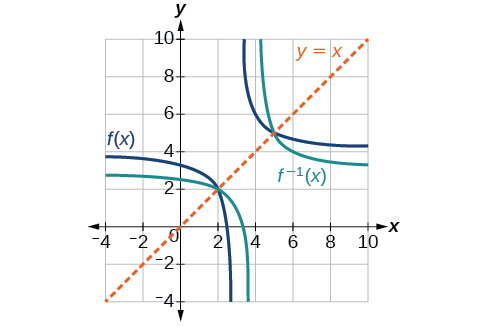# 3.7 Inverse functions  (Page 6/9)

 Page 6 / 9

Draw graphs of the functions and from [link] .Is there any function that is equal to its own inverse?

Yes. If $\text{\hspace{0.17em}}f={f}^{-1},\text{\hspace{0.17em}}$ then $\text{\hspace{0.17em}}f\left(f\left(x\right)\right)=x,\text{\hspace{0.17em}}$ and we can think of several functions that have this property. The identity function does, and so does the reciprocal function, because

$\frac{1}{\frac{1}{x}}=x$

Any function $\text{\hspace{0.17em}}f\left(x\right)=c-x,\text{\hspace{0.17em}}$ where $\text{\hspace{0.17em}}c\text{\hspace{0.17em}}$ is a constant, is also equal to its own inverse.

Access these online resources for additional instruction and practice with inverse functions.

Visit this website for additional practice questions from Learningpod.

## Key concepts

• If $\text{\hspace{0.17em}}g\left(x\right)\text{\hspace{0.17em}}$ is the inverse of $\text{\hspace{0.17em}}f\left(x\right),\text{\hspace{0.17em}}$ then $\text{\hspace{0.17em}}g\left(f\left(x\right)\right)=f\left(g\left(x\right)\right)=x.\text{\hspace{0.17em}}$ See [link] , [link] , and [link] .
• Only some of the toolkit functions have an inverse. See [link] .
• For a function to have an inverse, it must be one-to-one (pass the horizontal line test).
• A function that is not one-to-one over its entire domain may be one-to-one on part of its domain.
• For a tabular function, exchange the input and output rows to obtain the inverse. See [link] .
• The inverse of a function can be determined at specific points on its graph. See [link] .
• To find the inverse of a formula, solve the equation $\text{\hspace{0.17em}}y=f\left(x\right)\text{\hspace{0.17em}}$ for $\text{\hspace{0.17em}}x\text{\hspace{0.17em}}$ as a function of $\text{\hspace{0.17em}}y.\text{\hspace{0.17em}}$ Then exchange the labels $\text{\hspace{0.17em}}x\text{\hspace{0.17em}}$ and $\text{\hspace{0.17em}}\text{\hspace{0.17em}}y.\text{\hspace{0.17em}}\text{\hspace{0.17em}}$ See [link] , [link] , and [link] .
• The graph of an inverse function is the reflection of the graph of the original function across the line $\text{\hspace{0.17em}}y=x.\text{\hspace{0.17em}}$ See [link] .

## Verbal

Describe why the horizontal line test is an effective way to determine whether a function is one-to-one?

Each output of a function must have exactly one output for the function to be one-to-one. If any horizontal line crosses the graph of a function more than once, that means that $\text{\hspace{0.17em}}y$ -values repeat and the function is not one-to-one. If no horizontal line crosses the graph of the function more than once, then no $\text{\hspace{0.17em}}y$ -values repeat and the function is one-to-one.

Why do we restrict the domain of the function $\text{\hspace{0.17em}}f\left(x\right)={x}^{2}\text{\hspace{0.17em}}$ to find the function’s inverse?

Can a function be its own inverse? Explain.

Yes. For example, $\text{\hspace{0.17em}}f\left(x\right)=\frac{1}{x}\text{\hspace{0.17em}}$ is its own inverse.

Are one-to-one functions either always increasing or always decreasing? Why or why not?

How do you find the inverse of a function algebraically?

Given a function $\text{\hspace{0.17em}}y=f\left(x\right),\text{\hspace{0.17em}}$ solve for $\text{\hspace{0.17em}}x\text{\hspace{0.17em}}$ in terms of $\text{\hspace{0.17em}}y.\text{\hspace{0.17em}}$ Interchange the $\text{\hspace{0.17em}}x\text{\hspace{0.17em}}$ and $\text{\hspace{0.17em}}y.\text{\hspace{0.17em}}$ Solve the new equation for $\text{\hspace{0.17em}}y.\text{\hspace{0.17em}}$ The expression for $\text{\hspace{0.17em}}y\text{\hspace{0.17em}}$ is the inverse, $\text{\hspace{0.17em}}y={f}^{-1}\left(x\right).$

## Algebraic

Show that the function $\text{\hspace{0.17em}}f\left(x\right)=a-x\text{\hspace{0.17em}}$ is its own inverse for all real numbers $\text{\hspace{0.17em}}a.\text{\hspace{0.17em}}$

For the following exercises, find $\text{\hspace{0.17em}}{f}^{-1}\left(x\right)\text{\hspace{0.17em}}$ for each function.

$f\left(x\right)=x+3$

${f}^{-1}\left(x\right)=x-3$

$f\left(x\right)=x+5$

$f\left(x\right)=2-x$

${f}^{-1}\left(x\right)=2-x$

$f\left(x\right)=3-x$

$f\left(x\right)=\frac{x}{x+2}$

${f}^{-1}\left(x\right)=\frac{-2x}{x-1}$

$f\left(x\right)=\frac{2x+3}{5x+4}$

For the following exercises, find a domain on which each function $\text{\hspace{0.17em}}f\text{\hspace{0.17em}}$ is one-to-one and non-decreasing. Write the domain in interval notation. Then find the inverse of $\text{\hspace{0.17em}}f\text{\hspace{0.17em}}$ restricted to that domain.

$f\left(x\right)={\left(x+7\right)}^{2}$

domain of $f\left(x\right):\text{\hspace{0.17em}}\left[-7,\infty \right);\text{\hspace{0.17em}}{f}^{-1}\left(x\right)=\sqrt{x}-7$

$f\left(x\right)={\left(x-6\right)}^{2}$

$f\left(x\right)={x}^{2}-5$

domain of $\text{\hspace{0.17em}}f\left(x\right):\text{\hspace{0.17em}}\left[0,\infty \right);\text{\hspace{0.17em}}{f}^{-1}\left(x\right)=\sqrt{x+5}$

Given $\text{\hspace{0.17em}}f\left(x\right)=\frac{x}{2+x}\text{\hspace{0.17em}}$ and $\text{\hspace{0.17em}}g\left(x\right)=\frac{2x}{1-x}:$

1. Find $\text{\hspace{0.17em}}f\left(g\left(x\right)\right)\text{\hspace{0.17em}}$ and $\text{\hspace{0.17em}}g\left(f\left(x\right)\right).$
2. What does the answer tell us about the relationship between $\text{\hspace{0.17em}}f\left(x\right)\text{\hspace{0.17em}}$ and $\text{\hspace{0.17em}}g\left(x\right)?$

a. and $\text{\hspace{0.17em}}g\left(f\left(x\right)\right)=x.\text{\hspace{0.17em}}$ b. This tells us that $\text{\hspace{0.17em}}f\text{\hspace{0.17em}}$ and $\text{\hspace{0.17em}}g\text{\hspace{0.17em}}$ are inverse functions

f(x)=x/x+2 given g(x)=1+2x/1-x show that gf(x)=1+2x/3
proof
AUSTINE
sebd me some questions about anything ill solve for yall
how to solve x²=2x+8 factorization?
x=2x+8 x-2x=2x+8-2x x-2x=8 -x=8 -x/-1=8/-1 x=-8 prove: if x=-8 -8=2(-8)+8 -8=-16+8 -8=-8 (PROVEN)
Manifoldee
x=2x+8
Manifoldee
×=2x-8 minus both sides by 2x
Manifoldee
so, x-2x=2x+8-2x
Manifoldee
then cancel out 2x and -2x, cuz 2x-2x is obviously zero
Manifoldee
so it would be like this: x-2x=8
Manifoldee
then we all know that beside the variable is a number (1): (1)x-2x=8
Manifoldee
so we will going to minus that 1-2=-1
Manifoldee
so it would be -x=8
Manifoldee
so next step is to cancel out negative number beside x so we get positive x
Manifoldee
so by doing it you need to divide both side by -1 so it would be like this: (-1x/-1)=(8/-1)
Manifoldee
so -1/-1=1
Manifoldee
so x=-8
Manifoldee
Manifoldee
so we should prove it
Manifoldee
x=2x+8 x-2x=8 -x=8 x=-8 by mantu from India
mantu
lol i just saw its x²
Manifoldee
x²=2x-8 x²-2x=8 -x²=8 x²=-8 square root(x²)=square root(-8) x=sq. root(-8)
Manifoldee
I mean x²=2x+8 by factorization method
Kristof
I think x=-2 or x=4
Kristof
x= 2x+8 ×=8-2x - 2x + x = 8 - x = 8 both sides divided - 1 -×/-1 = 8/-1 × = - 8 //// from somalia
Mohamed
hii
Amit
how are you
Dorbor
well
Biswajit
can u tell me concepts
Gaurav
Find the possible value of 8.5 using moivre's theorem
which of these functions is not uniformly cintinuous on (0, 1)? sinx
which of these functions is not uniformly continuous on 0,1
solve this equation by completing the square 3x-4x-7=0
X=7
Muustapha
=7
mantu
x=7
mantu
3x-4x-7=0 -x=7 x=-7
Kr
x=-7
mantu
9x-16x-49=0 -7x=49 -x=7 x=7
mantu
what's the formula
Modress
-x=7
Modress
new member
siame
what is trigonometry
deals with circles, angles, and triangles. Usually in the form of Soh cah toa or sine, cosine, and tangent
Thomas
solve for me this equational y=2-x
what are you solving for
Alex
solve x
Rubben
you would move everything to the other side leaving x by itself. subtract 2 and divide -1.
Nikki
then I got x=-2
Rubben
it will b -y+2=x
Alex
goodness. I'm sorry. I will let Alex take the wheel.
Nikki
ouky thanks braa
Rubben
I think he drive me safe
Rubben
how to get 8 trigonometric function of tanA=0.5, given SinA=5/13? Can you help me?m
More example of algebra and trigo
What is Indices
If one side only of a triangle is given is it possible to solve for the unkown two sides?
cool
Rubben
kya
Khushnama
please I need help in maths
Okey tell me, what's your problem is?
NavinByByByByByBy